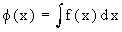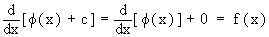Home MonkeyNotes Printable Notes Digital Library Study Guides Study Smart Parents Tips College Planning Test Prep Fun Zone Help / FAQ How to Cite New Title Request

CHAPTER 6 : INTEGRATION

We know that in algebra the operations of addition and subtraction, multiplication and division form reverse pairs of operations. In calculus the operations of derivative and integration (anti-derivative) are also thought of an inverse of one another. Thus they also form a reverse pair of operations and the rules for finding derivatives will be useful in establishing corresponding rules for finding anti-derivatives.

6.1 Anti - Derivatives ( Indefinite Integrals )

If a function f exists , for a given function f, such that ( x ) = f ( x ), then f is called anti-derivative of f.

An anti-derivative is also known as primitive or an indefinite integral.

Symbolically, this is written asand is read as " f ( x ) is an integral of f ( x )" w.r. to x .

Here f ( x ) is called the integrand and the process of finding the integral is called integration.

The above definition poses the following questions.

(1) Does every function poses an anti-derivative?

(2) Is an anti-derivative unique in case it exists?

(3) Is it always possible to find an anti-derivative?

The following answers are suggested :

(1) There exist a large number of functions possessing an anti-derivative, e.g. all continuous functions. In finding an anti-derivative, we shall always assume its existence. However every function need not posses an anti-derivative.Your browser does not support the IFRAME tag.

(2) Letso that
for any constant cso that f ( x ) + c is also an anti-derivative of f ( x ). This accounts for the name "Indefinite Integral".

(3) In the present chapter, we shall study several methods of finding anti-derivatives. This, however, does not ensure that anti-derivative of any given function can always be obtained.

Index

6.1 Anti-derivatives (indefinite Integral)
6.2 Integration Of Some Trignometric Functions
6.3 Methods Of Integration
6.4 Substitution And Change Of Variables
6.5 Some Standard Substitutions
6.6 Integration By Parts
6.7 Integration By Partial Fractions

Chapter 7